## December 6, 2009

### This Week’s Finds in Mathematical Physics (Week 285)

#### Posted by John BaezIn week285 of This Week’s Finds, discover the beauty of roots: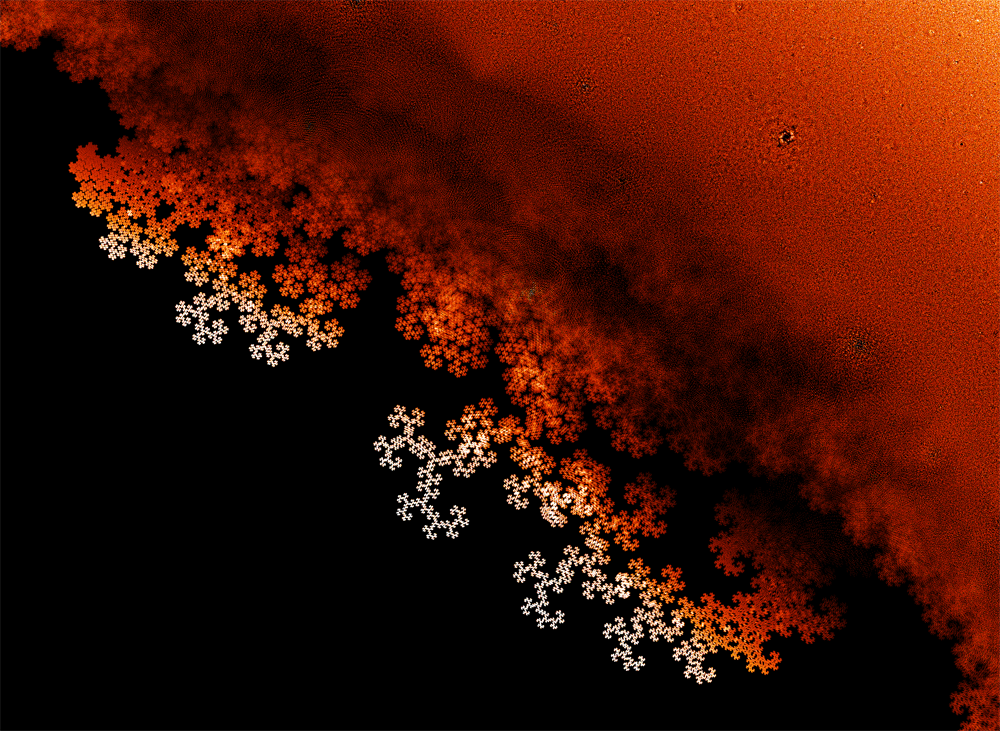Then hear about the second day’s talks on homotopy theory and higher algebraic structures at the AMS conference at U.C. Riverside. Learn about string topology, string connections, 2-gerbes, orbifolds, twisted K-theory, monoids of moduli spaces of manifolds, categorified quandles, long knots and Vassiliev theory, 3-categories for the working mathematician… and the blob complex!

This picture by Sam Derbyshire deserves to be bigger:Posted at December 6, 2009 2:38 AM UTC

TrackBack URL for this Entry:   https://golem.ph.utexas.edu/cgi-bin/MT-3.0/dxy-tb.fcgi/2125

### Re: This Week’s Finds in Mathematical Physics (Week 285)

Wow! Exquisite as those pictures by Christensen and Derbyshire are, what really gets me is that this is such an obvious thing to do, yet apparently no one had thought to do it before. (Or had they?)

It reminds me of something I read about the resurgence of interest in complex dynamics, in the late 1970s. (If I understand correctly, the field had been largely dormant since about 1920, when Fatou and Julia did their pioneering work.) A bunch of people, including Brooks, Matelski, Douady, Hubbard and Mandelbrot, began to study the iteration of quadratic maps, with the aid of the emerging computer technology.

Douady was working in Paris, close to the IHES. It must have been an amazing place and time. Deligne had solved the Weil conjectures not so long ago. And Douady… Douady was studying quadratic equations. There’s some interview with or about him, which I can’t find now, where he recalls someone asking “These quadratics — do you really hope to find anything new?”

And while I’m recalling anecdotes, I can’t resist adding this one, from Milnor’s book Dynamics in One Complex Variable.

Mandelbrot made quite good computer pictures [of the Mandelbrot set, about 1980], which seemed to show a number of isolated “islands”. Therefore, he conjectured that [the Mandelbrot set] has many distinct connected components. (The editors of the journal thought that his islands were specks of dirt, and carefully removed them from the pictures.)

Posted by: Tom Leinster on December 6, 2009 3:55 AM | Permalink | Reply to this

### Re: This Week’s Finds in Mathematical Physics (Week 285)

Tom wrote:

Exquisite as those pictures by Christensen and Derbyshire are, what really gets me is that this is such an obvious thing to do, yet apparently no one had thought to do it before. (Or had they?)

Dan first plotted those roots in late 2005 or early 2006. I put the pictures on my website in sci.math.research in January 2006 and got some replies pointing to earlier work. You’ll see other earlier work listed in the Mathworld article that I cited in week285.

So, Dan and Sam weren’t the first. But the pictures in that Mathworld article scarcely begin to reveal the full beauty of what’s going on! I think there’s a lot of room for exploration and ‘experimental mathematics’ here. Mathematicians should start making conjectures and proving theorems… but people who like to program should just dive in and try all sorts of stuff!

If anyone makes some nice pictures, please let me know.

Posted by: John Baez on December 6, 2009 6:02 PM | Permalink | Reply to this

### Re: This Week’s Finds in Mathematical Physics (Week 285)

Hi John,

so, basically your friend discovered the first fractal that was not generated by any kind of iterative process! WOW!

Posted by: Daniel de Franca MTd2 on December 6, 2009 3:56 AM | Permalink | Reply to this

### Re: This Week’s Finds in Mathematical Physics (Week 285)

That could be overstating it. The Cantor set can be described as the set of all real numbers in $[0, 1]$ that admit a ternary expansion consisting entirely of 0s and 2s. That’s not inherently iterative.

Sierpiński’s triangle can be viewed as Pascal’s triangle mod 2, and Pascal’s triangle can be defined non-iteratively by the usual factorial formula for binomial coefficients.

Even Julia sets can be defined non-iteratively: for a complex rational function $f$ of degree at least two, the Julia set $J$ of $f$ is the smallest closed subset of $\mathbb{C} \cup \{\infty\}$ satisfying $f^{-1}J = J$ and containing at least three points.

Nevertheless, all these examples can be defined iteratively.

Posted by: Tom Leinster on December 6, 2009 5:02 AM | Permalink | Reply to this

### Re: This Week’s Finds in Mathematical Physics (Week 285)

Another thing to keep in mind is that Christensen’s and Derbyshire’s pictures are all of finite spaces. I’m not just saying that the computer-generated pictures are finite (as any collection of pixels is). I mean that the mathematical objects are themselves finite.

So I don’t know in what sense they could be called fractals. Being finite spaces, their dimension in any of the usual senses is 0. But they certainly look like fractals, so perhaps there’s something to be said.

Posted by: Tom Leinster on December 6, 2009 5:24 AM | Permalink | Reply to this

### Re: This Week’s Finds in Mathematical Physics (Week 285)

Well, any fractal displayed in a computer display is finite. But notice that here what improves definition is not the number the number the number of iterations but the range of the integers of the polynomial.

Regarding the iterative nature, I was thinking not by the definition but on how it was generated. But seeing by the generative aspect, I think this case is closer to the case of Sierpiński’s triangle and the newton binomial. Except that you have to squeeze the Pascal Triangle as one increases the number of rows of the polynomial.

BTW Tom, I guess you can indeed see clearly 2 regions that apparently display a fractal nature. Take a look at the 3rd and 4th picture, from top to bottom. One region is contained in big the shining ring: the hole distribution resembles a cantor set. The second region is in the 4th pic, bellow the little black hole at pi/2, you can see a straight line containing something also similar to a cantor set.

Notice that at least the graph with zeros of the Jones polynomial also displays something like a ring cantor.

Posted by: Daniel de França MTd2 on December 6, 2009 12:09 PM | Permalink | Reply to this

### Re: This Week’s Finds in Mathematical Physics (Week 285)

I found this book, Peter Borwein, Computational Excursions in Analysis and Number Theory. He starts the chapter by writing “The location of the zeros of Littlewood polynomials and related classes of low height polynomials is subtle and interesting. The zeros cluster hearvily around the unit circle and appear to form a set with fractal boundary”. Right bellow he plots the same polynomial graph with solutions.

This book is from 2002, and it looks like this weird picture is not that new. All that chapter is devoted to find the properties of this plot.

Posted by: Daniel de França MTd2 on December 6, 2009 1:19 PM | Permalink | Reply to this

### Re: This Week’s Finds in Mathematical Physics (Week 285)

Daniel wrote:

I found this book, Peter Borwein, Computational Excursions in Analysis and Number Theory.

Yeah, that’s one of references in the Mathworld article I pointed to. But I haven’t found much detailed study of the more interesting patterns clearly visible in these sets. There are lots of theorems waiting to proved, and papers waiting to be written!

Posted by: John Baez on December 6, 2009 11:11 PM | Permalink | Reply to this

### Re: This Week’s Finds in Mathematical Physics (Week 285)

Tom wrote:

Another thing to keep in mind is that Christensen’s and Derbyshire’s pictures are all of finite spaces.

But they certainly look like fractals, so perhaps there’s something to be said.

I don’t remember the details, but I heard a talk about this work dealing with fractal-like structure in paintings. They have a way of quantifying fractal-like behavior at different finite scales, which one could presumably apply to the pictures here.

Posted by: Mark Meckes on December 7, 2009 4:41 PM | Permalink | Reply to this

### Re: This Week’s Finds in Mathematical Physics (Week 285)

What a curious paper. If I’ve got it right, they’re exploring the idea that mathematical invariants might be used to tell who a painting’s by — and in particular, whether it’s by Jackson Pollock. That’s not their idea; indeed, they provide evidence that it’s harder than had been suggested.

Posted by: Tom Leinster on December 8, 2009 11:21 PM | Permalink | Reply to this

### Re: This Week’s Finds in Mathematical Physics (Week 285)

Yes, that’s the idea. The original work made a fairly big splash at the time; the paper I linked to is at least the third paper on the subject published in Nature.

The first-named author is a grad student (or was at the time the paper was written). Apparently she was preparing a seminar talk on the original paper about fractal analysis of Pollock paintings, found some of the arguments wanting, and thus this paper came to be written.

Posted by: Mark Meckes on December 9, 2009 12:44 AM | Permalink | Reply to this

### Re: This Week’s Finds in Mathematical Physics (Week 285)

3-categories for the working mathematician

I can't figure out what the URI should really be.

Posted by: Toby Bartels on December 6, 2009 4:34 AM | Permalink | Reply to this

### Re: This Week’s Finds in Mathematical Physics (Week 285)

Whoops! I don’t actually have a video for this talk. I think the speaker didn’t want to be videotaped.

Posted by: John Baez on December 6, 2009 6:32 AM | Permalink | Reply to this

### Re: This Week’s Finds in Mathematical Physics (Week 285)

A long knot is a curve in R3 that goes on forever and is a vertical straight line outside some compact set.

In other words, a long knot is a 1-strand tangle.

These are more like the knots in ordinary life; it's always a little disconcerting to tell people that mathematicians' knots are closed up end to end.

Posted by: Toby Bartels on December 6, 2009 4:37 AM | Permalink | Reply to this

### Re: This Week’s Finds in Mathematical Physics (Week 285)

In other words, a long knot is a 1-strand tangle.

Exactly. And more interesting to me, some invariants behave a lot better if you think of them as invariants of (1,1)-tangles instead of as invariants of (0,0)-tangles. Jones/Bracket should be thought of as on (0,0)-tangles of course, but Alexander should be thought of as an invariant on (1,1)-tangles.

Posted by: John Armstrong on December 6, 2009 5:00 AM | Permalink | Reply to this

### Re: This Week’s Finds in Mathematical Physics (Week 285)

Toby wrote:

In other words, a long knot is a 1-strand tangle.

Yeah, I don’t know why more people don’t come out and say that…

… and I really don’t know why I didn’t come out and say that.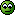Part of the reason must be that people use certain mathematical technologies when dealing with long knots, which seem to lend themselves to their description as infinitely long curves in $\mathbb{R}^3$.

But, I’ve fixed week285 based on your suggestion.

Posted by: John Baez on December 6, 2009 11:04 PM | Permalink | Reply to this

### Re: This Week’s Finds in Mathematical Physics (Week 285)

But I don't think that this phrasing

If you know about tangles, a long knot is just a tangle with one strand coming in on top and one coming out at bottom.

is quite accurate. A $(1,1)$-tangle (if that's the right notation) consists of a long knot and any number (perhaps none) of ordinary knots linked (perhaps trivially) together.

Posted by: Toby Bartels on December 7, 2009 12:18 AM | Permalink | Reply to this

### Re: This Week’s Finds in Mathematical Physics (Week 285)

Good point. I was trying to correct your phrasing, which didn’t make it clear that we want one strand coming in and one going out — instead of, say, two in and none out.

I think I’ve got it right now!

Posted by: John Baez on December 7, 2009 12:56 AM | Permalink | Reply to this

### Re: This Week’s Finds in Mathematical Physics (Week 285)

I think I’ve got it right now!

Agreed.

Posted by: Toby Bartels on December 7, 2009 9:21 PM | Permalink | Reply to this

### Long Line tied in knots…Re: This Week’s Finds in Mathematical Physics (Week 285)

That’s a relief. I flinched at first, thinking that this meant a knot tied on the Long Line.

In topology, the long line (or Alexandroff line) is a topological space analogous to the real line, but much longer. Because it behaves locally just like the real line, but has different large-scale properties, it serves as one of the basic counterexamples of topology.

Posted by: Jonathan Vos Post on December 8, 2009 7:01 PM | Permalink | Reply to this

### Re: This Week’s Finds in Mathematical Physics (Week 285)

So, where’s the almond blot? (I’m not being entirely facetious; it’s been known to turn up between attracting basins for various Newton-Raphson itterations, of all places.)

I also can’t help noticing that, if $P(x),Q(x)$ have $\pm 1$ coefficients, and $Q$ is of degree $d-1$, then $Q(x) P(x^d)$ also has coefficients $\pm 1$. That would be more than enough to describe the cloudiness of the picture near the unit circle: it’ll have all the $d^{th}$ roots of simpler roots — so a copy of the larger pattern repeating $d$ times, roughly a factor of $d$ closer to the circle — although with the polynomials here, $d$ or the degree of $P$ will be small, so it’s not a good model for “small” pictures like this. In a correlative direction, any guess about how many of these polynomials are irreducible?

Posted by: some guy on the street on December 6, 2009 4:48 AM | Permalink | Reply to this

### Re: This Week’s Finds in Mathematical Physics (Week 285)

So, where’s the almond blot?

The “mandelfleck”?

Posted by: John Armstrong on December 6, 2009 5:06 AM | Permalink | Reply to this

### Re: This Week’s Finds in Mathematical Physics (Week 285)

I’m confused. Is ‘almond speck’ supposed to mean ‘Mandelbrot set’? I feel like I’m not getting some joke. Is ‘almond speck’ a deliberate or accidental mistranslation of ‘Mandelbrot’, meaning ‘almond bread’? I can imagine almond speck, but that would be something else.

Posted by: John Baez on December 6, 2009 7:07 PM | Permalink | Reply to this

### Re: This Week’s Finds in Mathematical Physics (Week 285)

well, that and it does look kinda blot-like to me… lots of people have played Rorschach games with them, anyways.

Posted by: some guy on the street on December 6, 2009 9:27 PM | Permalink | Reply to this

### Re: This Week’s Finds in Mathematical Physics (Week 285)

Okay. Anyway, your actual mathematical idea sounds very interesting! We need mechanisms like this to explain the patterns in the pictures. There could be one or many such mechanisms at work… to spot them, it would be nice if you could click on a dot and see which polynomial it’s a root of! Or something like that.

Posted by: John Baez on December 6, 2009 10:43 PM | Permalink | Reply to this

### Re: This Week’s Finds in Mathematical Physics (Week 285)

Okay, to be a little more serious.

If “almond blot” means something like the Mandelbrot’s black snowman, then I’m not certain you’ll find one.

If I remember Yorke’s class correctly, the reason you see it is because it’s characteristic of locally quadratic behavior. It’s the stretch-and-fold of the quadratic function going up and back down that leads to chaotic behavior. Most maps are conjugate to this behavior – at least approximately – right near a local maximum or minimum inside the basin of an attractor.

If this fractal didn’t arise from iterating a map, then its behavior may be sufficiently distinct from such quadratic stretching-and-folding that it actually doesn’t get any snowmen at all.

Posted by: John Armstrong on December 7, 2009 3:08 AM | Permalink | Reply to this

### Re: This Week’s Finds in Mathematical Physics (Week 285)

The pictures on week285 are all low-resolution or partial versions of Sam Derbyshire’s original image. The original is available as a 90-megabyte file here.

Some of my Windows software is unable to display such a large file — it claims the file is corrupted. But irfanview can handle it. Slow, though!

Posted by: John Baez on December 6, 2009 7:01 PM | Permalink | Reply to this

### Re: This Week’s Finds in Mathematical Physics (Week 285)

I can think of a true first-order sentence involving quandles that doesn’t seem provable from the axioms (indeed, I don’t think it’s expressible as an equation), which is that, for any g, h in the quandle, there’s another element of the quandle, call it “gh,” such that

g > (h > k) = gh > k

for all k in the quandle. This is obvious if you write it out in the underlying group.

But gh (as an element of the group) can’t necessarily be written in terms of quandle elements and operations, since it might lie in a different conjugacy class than both g and h.

Of course this can’t directly be turned into an equation, but it seems like the major stumbling block in trying to prove that the quandle relations you give are complete.

Posted by: harrison on December 7, 2009 2:39 AM | Permalink | Reply to this

### Re: This Week’s Finds in Mathematical Physics (Week 285)

Harrison wrote:

I can think of a true first-order sentence involving quandles that doesn’t seem provable from the axioms (indeed, I don’t think it’s expressible as an equation)…

Nice! And here’s a simpler one:

$\exists x \forall y$   $x \gt y = y$

It’s true thanks to $x = 1$.

This is why my question concerned the ‘purely equational’ theory of quandles. This uses a form of logic that’s weaker than full-fledged first order logic. All you’re allowed is axioms of the form

$\forall x_1 \cdots \forall x_n f(x_1, \dots, x_n) = g(x_1, \dots, x_n)$

where $f$ and $g$ are built using function symbols… which in this case are the quandle operations $\gt$ and $\lt$.

‘Purely equational’ logic is formalized in universal algebra, first by Birkhoff’s varieties and nowadays by Lawvere theories.

You can take the Lawvere theory of groups and construct the ‘subtheory’ generated by the operations of left and right conjugation:

$g \gt h = g h g^{-1}$

$h \lt g = g^{-1} h g$

And then you can ask if all the equations in this subtheory follow from the equations in the definition of quandle. Everyone thinks it’s true… but I’ve never seen a proof!

Posted by: John Baez on December 7, 2009 3:11 AM | Permalink | Reply to this

### Re: This Week’s Finds in Mathematical Physics (Week 285)

And then you can ask if all the equations in this subtheory follow from the equations in the definition of quandle. Everyone thinks it’s true… but I’ve never seen a proof!

It’s been a while but I’m almost positive that’s in one of Joyce’s first two papers.

Posted by: John Armstrong on December 7, 2009 3:39 AM | Permalink | Reply to this

### Re: This Week’s Finds in Mathematical Physics (Week 285)

Here’s another example of the same sort of question. Start with the Lawvere theory of rings and define an operation

$[x,y] = x y - y x$

What’s a complete set of equational laws obeyed by this operation? Everyone thinks they know the answer to this one, and in this case I think it’s easy to prove they’re right. (A little challenge!)

Or: take the Lawvere theory of rings and define an operation

$x \circ y = x y + y x$

What’s a complete set of equational laws obeyed by this operation? This is famously tricky.

From week192:

Two of the identities are obvious:

$1 \circ X = X$

$X \circ Y = Y \circ X$

The next one is less obvious:

$X \circ ((X \circ X) \circ Y) = (X \circ X) \circ (X \circ Y)$

At this point, Pascual Jordan quit looking for more and made these his definition of what we now call a “Jordan algebra”:

12) Pascual Jordan, Ueber eine Klasse nichtassociativer hyperkomplexer Algebren, Nachr. Ges. Wiss. Goettingen (1932), 569-575.

He wrote this paper while pondering the foundations of quantum theory, since bounded self-adjoint operators on a Hilbert space represent observables, and they’re closed under the product $X \circ Y = X Y + Y X$.

Later, with Eugene Wigner and John von Neumann, he classified all finite-dimensional Jordan algebras that are “formally real”, meaning that a sum of terms of the form $X \circ X$ is zero only if each one is zero. This condition is reasonable in quantum mechanics, because observables like $X \circ X$ are “positive”. It also leads to a nice classification, which I described in “week162”.

Interestingly, one of these formally real Jordan algebras doesn’t sit inside an associative algebra: the “exceptional Jordan algebra”, which consists of all $3 \times 3$ hermitian matrices with octonion entries.

This algebra has lots of nice properties, and it plays a mysterious role in string theory and some other physics theories. This is the main reason I’m interested in Jordan algebras, but I’ve said plenty about this already; now I want to focus on something else.

Namely: did Jordan find all the identities?

More precisely: if we set $X \circ Y = X Y + Y X$$, can all the identities satisfied by this operation in every associative algebra be derived from the above 3 and the fact that this operation is linear in each slot?

This was an open question until 1966, when Charles M. Glennie found the answer is no.

It's a bit like Tarski's "high school algebra problem", where Tarksi asked if all the identities involving addition, multiplication and exponentiation which hold for the positive the natural numbers follow from the ones we all learned in high school. Here too the answer is no - see "week172" for details. That really shocked me when I heard about it! Glennie's result is less shocking, because Jordan algebras are less familiar... and the Jordan identity is already pretty weird, so maybe we should expect other weird identities.

It's easiest to state Glennie's identity with the help of the "Jordan triple product"

$

$\{X,Y,Z\} = (X \circ Y) \circ Z + (Y \circ Z) \circ X - (Z \circ X) \circ Y$$

Here it is:

$

$2\{\{Y, \{X,Z,X\},Y\}, Z, X\circ Y\}$$\text{}$$- \{Y, \{X,\{Z,X \circ Y,Z\},X\}, Y\}$$\text{}$$-2\{X \circ Y, Z, \{X,\{Y,Z,Y\},X\}\}$$\text{}$$+ \{X, \{Y,\{Z,X \circ Y,Z\},Y\}, X\} = 0$\$

Blecch! It makes you wonder how Glennie found this, and why.

I don’t know the full story, I know but Glennie was a Ph.D. student of Nathan Jacobson, a famous algebraist and expert on Jordan algebras. I’m sure that goes a long way to explain it. He published his result here:

13) C. M. Glennie, Some identities valid in special Jordan algebras but not in all Jordan algebras, Pacific J. Math. 16 (1966), 47-59.

Well, I’m afraid the title of the paper gives that away: in addition to the above identity of degree 8, Glennie also found another. In fact there turn out to be infinitely many identities that can’t be derived from the previous ones using the Jordan algebra operations.

As far as I can tell, the full story was discovered only in the 1980s. Let me quote something by Murray Bremner. It will make more sense if you know that the identities we’re after are called “s-identities”, since they hold in “special” Jordan algebras: those coming from associative algebras. Here goes:

Efim Zelmanov won the Fields Medal at the International Congress of Mathematicians in Zurich in 1994 for his work on the Burnside Problem in group theory. Before that he had solved some of the most important open problems in the theory of Jordan algebras. In particular he proved that Glennie’s identity generates all s-identities in the following sense: if G is the T-ideal generated by the Glennie identity in the free Jordan algebra FJ(X) on the set X (where X has at least 3 elements), then the ideal S(X) of all s-identities is quasi-invertible modulo G (and its homogeneous components are nil modulo G) [….] Roughly speaking, this means that all other s-identities can be obtained by substituting into the Glennie identity, generating an ideal, extracting n-th roots, and summing up.

This is a bit technical, but basically it means you need to expand your arsenal of tricks a bit before Glennie’s identity gives all the rest. The details can be found in Theorem 6.7 here:

14) Kevin McCrimmon, Zelmanov’s prime theorem for quadratic Jordan algebras, Jour. Alg. 76 (1982), 297-326.

and I got the above quote from a talk by Bremner:

15) Murray Bremner, Using linear algebra to discover the defining identities for Lie and Jordan algebras, available at http://web.archive.org/web/20030324024322/http://math.usask.ca/~bremner/research/colloquia/calgarynew.pdf

Posted by: John Baez on December 7, 2009 3:52 AM | Permalink | Reply to this

### Re: This Week’s Finds in Mathematical Physics (Week 285)

Okay, I’m no logician, but if we add in some first-order sentences with one existential quantifier, that correspond to closure, inverse, and identity in the underlying group (this is highly doable), can we get… I guess what we want is the Lawvere theory of groups restricted to perfect groups (since we can only recover the underlying group up to its group of inner automorphisms)? I guess from there it might be possible to say something of interest.

Posted by: harrison on December 7, 2009 5:13 AM | Permalink | Reply to this

### Re: This Week’s Finds in Mathematical Physics (Week 285)

I’m a bit out of my league here, but a fractal can be constructed by taking an initial set of points and applying an affine transformation. It’s how IFS fractals are made anyway I think.

It would then seem that we can do an affine transformation of a set of solutions to arrive at new solutions. But why the particular affine transformation producing this fractal?

Remembering that the roots of any polynomial may be written in terms of multivariate hypergeometric functions it seems to me that doi:10.1016/j.bulsci.2006.12.004 among others are touching on this topic.

Posted by: Robert on December 7, 2009 12:26 PM | Permalink | Reply to this

### Re: This Week’s Finds in Mathematical Physics (Week 285)

A few people have used the word “fractal” in connection with these pictures. This makes me rather puzzled.

On the one hand, the spaces portrayed are finite. John wrote, in TWF:

Let’s define the set $C(d,n)$ to be the set of all roots of all polynomials of degree [at most] $d$ with integer coefficients ranging from $-n$ to $n$.

These spaces $C(d, n)$ are finite. All the pictures in TWF are of $C(d, n)$ (or a subset thereof) for some choice of $d$ and $n$; so all the pictures are of finite spaces. To be clear: the pictures aren’t finite approximations to infinite spaces, as many pictures of fractals are. The spaces being portrayed really are finite.

Now, from most points of view finite spaces are very boring. In particular, they have dimension $0$ in just about any sense of “dimension” you like. One characteristic property of fractals (a word for which there’s no agreed definition) is that the Hausdorff dimension is greater than the topological dimension. But that’s not the case here. Another general feature is that as you zoom in, you keep seeing more detail. Again, that’s not the case here.

So, it seems hard to say that these spaces are in any sense fractals.

On the other hand, look at them! They have a clear resemblance to all the things we call fractals. So, are these spaces $C(d, n)$ approximations to some fractal space? John wrote:

Clearly $C(d,n)$ gets bigger as we make either $d$ or $n$ bigger. It becomes dense in the complex plane as $n \to \infty$, as long as $d \geq 1$ [should be $d \geq 2$?]. We get all the rational complex numbers if we fix $d \geq 1$ [2?] and let $n \to \infty$, and all the algebraic complex numbers if let both $d,n \to \infty$.

But what happens if we fix $n$ and let $d \to \infty$? Write $C(\infty, n) = \bigcup_{d = 0}^\infty C(d, n).$ E.g. $C(\infty, 1)$ is the set of all $z \in \mathbb{C}$ satisfying some equation such as $z^3 + z^5 = z^4 + z^7 + z^{11}.$ I can’t figure out anything at all about this set. Is it dense in $\mathbb{C}$? Bounded? Unbounded? Is it, in fact, some fractal space of which the finite spaces $C(d, n)$ are approximations?

John mentioned Odlyzko and Poonen’s result on polynomials with coefficients in $\{0, 1\}$ (as opposed to $\{-1, 0, 1\}$, the example just mentioned). There, you don’t get anything like all of $\mathbb{C}$; in fact, all the roots lie in a certain annulus. But that asymmetric constraint on the coefficients strikes me as changing the character of the problem.

Posted by: Tom Leinster on December 7, 2009 1:30 PM | Permalink | Reply to this

### Re: This Week’s Finds in Mathematical Physics (Week 285)

Hi Tom,

as far as I can see this is indeed an approximation to an infinite object. Note that what matters here it is the degree of the polynomial. The biggest the degree of the more accurate the picture will be. The whole picture comes if you use all polynomials up to an infinite degree.

Take a look at the reference 5 provided by John and you will see that the picture becomes more accurate if you sample a larger number of polynomials/roots.

Posted by: Daniel de Franca MTd2 on December 7, 2009 2:35 PM | Permalink | Reply to this

### Re: This Week’s Finds in Mathematical Physics (Week 285)

You’re right, Daniel, these finite spaces $C(d, n)$ (for fixed $n$ and varying $d)$ are indeed approximations to an infinite space, namely $C(\infty, n)$. That’s the easy bit: $C(\infty, n)$ was defined the way it was in order to make this true.

The hard bit is: what does $C(\infty, n)$ look like?

Posted by: Tom Leinster on December 7, 2009 5:45 PM | Permalink | Reply to this

### Re: This Week’s Finds in Mathematical Physics (Week 285)

I agree that some kind of infinite limit of these spaces would be easier and more interesting than the finite versions. (Interesting how infinity is a simpler number than 24, say.) You can understand the limit in the sense of unions of subsets of the complex plane, but another way might be as measures on the complex plane, perhaps suitably normalized as the parameters go to infinity. I bring this up because some of the pictures look like point clouds, which measure theory is probably well-suited to the analysis of.

Posted by: James on December 8, 2009 2:38 AM | Permalink | Reply to this

### Re: This Week’s Finds in Mathematical Physics (Week 285)

Posted by: Tom Leinster on December 8, 2009 11:26 PM | Permalink | Reply to this

### Re: This Week’s Finds in Mathematical Physics (Week 285)

The hard bit is: what does $C(\infty,n)$ look like?

And in particular, is it a fractal?

Posted by: Toby Bartels on December 7, 2009 9:25 PM | Permalink | Reply to this

### Re: This Week’s Finds in Mathematical Physics (Week 285)

Well, this set does not contain any point within $\{ |z| < 1/2 \}$ other than $0$. Proof: Let $|z| < 1/2$, with $z \neq 0$, and suppose that $z$ is a zero of $\sum e_k z^k$ with $e_k \in \{-1, 0 ,1\}$. After dividing by a power of $z$, we may assume that $e_0 \neq 0$.

Then $\sum e_k z^k = e_0 + \sum_{k \geq 1} e_k z_k$.

The second term is at most $\sum_{k \geq 1} |z|^k < 1$, so the left hand side cannot be zero.

Posted by: David Speyer on December 7, 2009 2:42 PM | Permalink | Reply to this

### Re: This Week’s Finds in Mathematical Physics (Week 285)

Ah, good. And if I’m not mistaken, the same argument shows that $0$ is an isolated point of $C(\infty, n)$ for any $n$. Hence $C(\infty, n)$ is never dense in $\mathbb{C}$.

(More precisely, if $0 \lt |z| \lt 1/(n + 1)$ then $z$ is not in $C(\infty, n)$.)

Posted by: Tom Leinster on December 7, 2009 5:50 PM | Permalink | Reply to this

### Re: This Week’s Finds in Mathematical Physics (Week 285)

It’s very nice to ‘trap’ the set $C(\infty,n)$ inside an annulus as Tom and David have just done. (They showed that points with $|z| \lt 1/(n+1)$ can’t be in this set, but if $z$ is this set so is $z^{-1}$.)

As I mentioned, Odlyzko and Poonen also trap their set — the roots of polynomials whose coefficients are $0$ or $1$ — in an annulus… but they did better: they succeeded in trapping it between two subtler curves. So, we can imagine people improving David’s bounds…

… but I what I’d like even more is an explanation of the beautiful patterns in sets of this type. Maybe we need results like: ‘if $z$ is in our set, so is $f(z)$.’ Some guy on the street has already proved a result like this for Sam Derbyshire’s set, but this result is not enough to explain the beautiful stuff going on here:So, there are probably other results of the same type — or perhaps ‘if $z$ is in our set and also in some set $X$, so is $f(z)$.’ Can anyone find some?

(Warning: Derbyshire’s set shown above consists of roots of polynomials with coefficients $1$ or $-1$, not $0$.)

Posted by: John Baez on December 7, 2009 6:20 PM | Permalink | Reply to this

### Re: This Week’s Finds in Mathematical Physics (Week 285)

I think John Baez was wise in that not once does he use the word “fractal”. Your earlier comments on non-iterative ways to define certain fractals was very interesting.

As for “fractal-like” if a subset A of C(d,n) could be related to another subset B of C(d,n) such that B is an affine transformation of A, then we have the building blocks for an IFS fractal. For this to result in the structures seen here, we would need several affine transformation which could be applied repeatedly. Now many fractals “look alike”, but the above picture is still quite similar to the “dragon curve” which is represented by an especially simple set of iterations in the complex plane:

f1(z) = (1+i)z/2
f2(z) = 1 - (1-i)z/2

My previous claim would then be: For any root s in a subset S of C(d,n) we have that:

1) f1(s) and/or f2(s) is contained in C(d,n)

2) f1(s) and/or f2(s) is contained in S.

Claim 1 ensure that the transformations produce new points and claim 2 that the transformations can be applied repeatedly. The finite size of the set means these claims can’t always hold, but they need only hold “most of the time” to produce the “fractal” patterns seen.

source: Wikipedia: Dragon Curve.

Posted by: Robert on December 7, 2009 2:49 PM | Permalink | Reply to this

### Re: This Week’s Finds in Mathematical Physics (Week 285)

This is an excellent strategy, Robert — much better than what I just suggested. Someone should find two functions $f_1$ and $f_2$ that do what you suggest and explain the dragonlike curve here:These functions don’t necessarily need to be affine!

If I didn’t have way too much fun stuff to do already, I’d instantly launch a Polymath Project and get different teams of people working to explain different aspects of Derbyshire’s set! It seems tailor-made for a ‘divide and conquer’ strategy.

Posted by: John Baez on December 7, 2009 6:34 PM | Permalink | Reply to this

### Re: This Week’s Finds in Mathematical Physics (Week 285)

The image is also rich in dragon curves, or self similar structures. Note specially the lighter colored horns, and you can see that.

Also, in this pic, see that all brighter regions, beginning in the place full of dragons until the x axis have that kind of fractal. It seems that they roughly follow the Lindenmayer system, as it is described in the Dragon Curve, where in the case of this polynomial picture, the angle in the construction is about twice the one in the origin.

Also, it seems as one aproaches 90º, the dragon turns inside out, and “becomes “something similar to a thick spiral that cannot “enter black zone.

So, I cannot see several different patterns, but just one… That is dragons that crouch so much that turns its inside out depending in the angle to the origin.

Posted by: Daniel de França MTd2 on December 7, 2009 8:47 PM | Permalink | Reply to this

### Re: This Week’s Finds in Mathematical Physics (Week 285)

In the big picture, note that there is an exact symmetry of the kind z=1/z around the bright ring. You can see the same dragons outside the ring that are inside.

And the dependence of that angle is more like 90º-2*angle.

Posted by: Daniel de França MTd2 on December 7, 2009 9:01 PM | Permalink | Reply to this

### Re: This Week’s Finds in Mathematical Physics (Week 285)

Daniel wrote:

In the big picture, note that there is an exact symmetry of the kind $z=1/z$ around the bright ring.

Yup. Sam’s picture shows all roots of all polynomials of degree $\le 24$ with coefficients $1$ or $-1$. As I already pointed out, it’s easy to see that if $z$ is a root of a polynomial of this sort, so is $1/z$.

(Not so easy? Okay, then it’s a fun puzzle!)

It’s also easy to see that if $z$ is a root of a polynomial of this sort, so are $-z$ and $z^*$.

Combining these ideas, we see his picture actually has at least an 8-element symmetry group.

But say we instead work with the set $C(\infty,n)$, drawn by Dan Christensen for $n = 5$: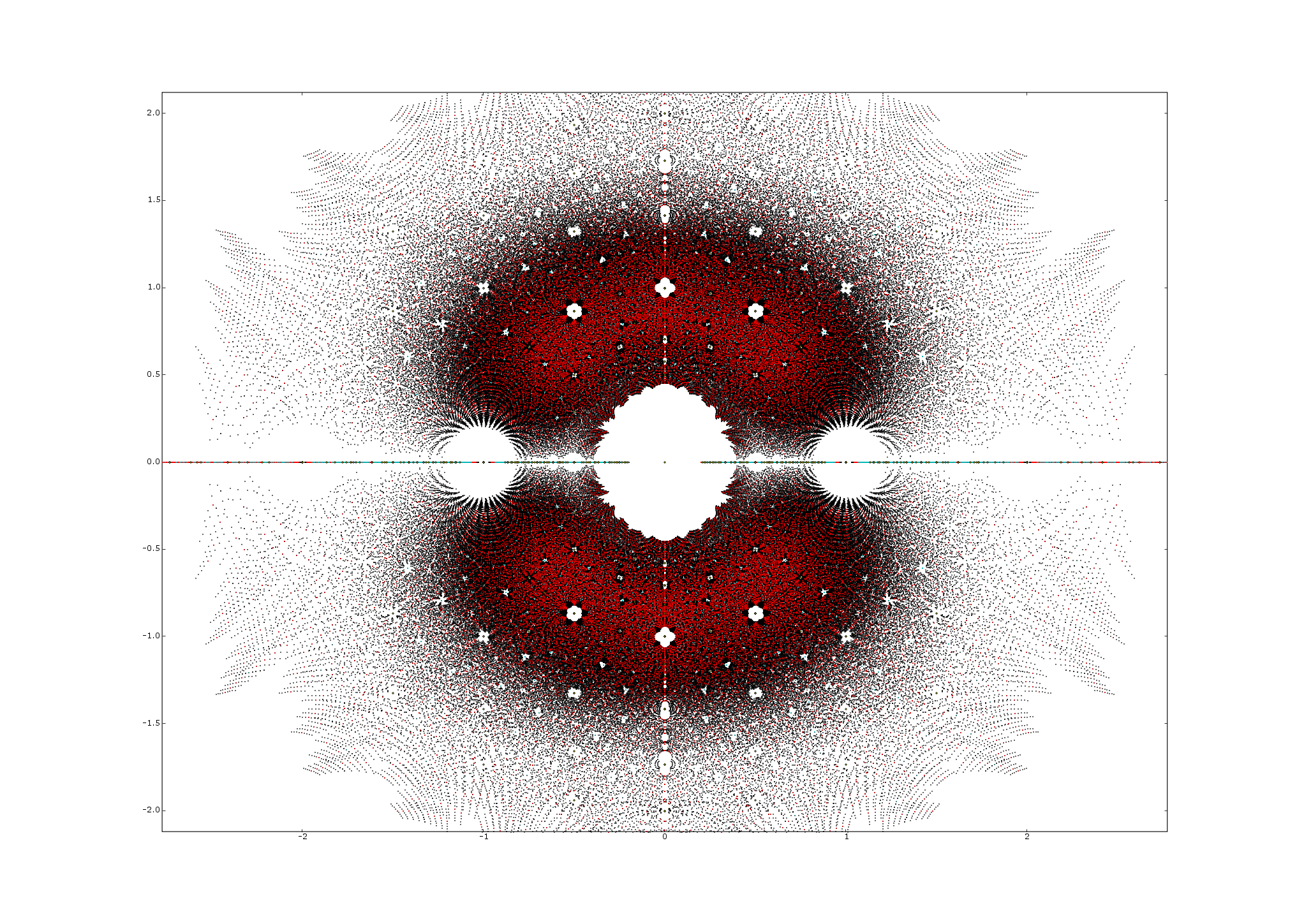Remember, this is the set of all roots of all polynomials with integer coefficients ranging from $-n$ to $n$. This lets us play even more tricks!

If $z$ is in this set, then we have $P(z) = 0$ for some polynomial $P$ of this sort, so we have $P(u^p) = 0$ whenever $u^p = z$. But as a function of $u$, $P(u^p)$ is again a polynomial of this sort. So all the $p$th roots of $z$ are again in the set!

An example should make this crystal clear: if

$z^4 - 2z^3 + z + 1 = 0$

then $z \in C(\infty,2)$. But then

$u^{12} - 2u^9 + u^3 + 1 = 0$

whenever $u^3 = z$. So then $u \in C(\infty,2)$.

I bet these are just the tips of the iceberg when it comes to the ‘hidden symmetries’ of the sets we’re looking at. Someone should find more! A free coffee for the best!

Posted by: John Baez on December 7, 2009 9:35 PM | Permalink | Reply to this

### Re: This Week’s Finds in Mathematical Physics (Week 285)

Your use of $n$ in “$n$th root” wasn’t ideal as you already use $n$ to bound the coefficients, especially as you then choose $n = 2$, which happens to bound the coefficients in the example.

Posted by: David Corfield on December 8, 2009 8:57 AM | Permalink | Reply to this

### Re: This Week’s Finds in Mathematical Physics (Week 285)

Whoops! I’ll use my superpowers to go back in time and say “$p$th root” instead. I really want people to understand what I wrote and come up with many more interesting statements of the form “if $z \in C(\infty,n)$ then $u \in C(\infty,n)$.”

Note: there’s something vaguely Galoisian about saying that if $z$ is in some set, so are all its $p$th roots, and the ‘symmetry’ this gives our set. I’m not quite sure what I mean…

Posted by: John Baez on December 8, 2009 9:35 AM | Permalink | Reply to this

### Re: This Week’s Finds in Mathematical Physics (Week 285)

You’d kinda think that the unit circle is acting as an ‘attractor’ to the constructions which stay within the set and which aren’t periodic. It wouldn’t surprise me if you had all the symmetries already.

Posted by: David Corfield on December 8, 2009 9:51 AM | Permalink | Reply to this

### Re: This Week’s Finds in Mathematical Physics (Week 285)

David wrote:

Well, I’ll only be satisfied when I see the explanation for patterns like this:This sort of thing arises naturally from an “IFS”, or “iterated function system” for those of us who hate acronyms. The idea is to look for a rule roughly like:

“If $z$ is in the set then $f(z)$ and $g(z)$ are in the set.”

or even:

“If $z$ is in the set then $f(z)$ or $g(z)$ is in the set.”

as long as the probabilities for the two outcomes are ‘well-behaved’ in some sense.

Then, for a very simple choice of $f$ and $g$, and letting our set contain some points to start with as a kind of ‘seed’, we easily grow sets like the Heighway dragon:You should like this, because it’s coalgebraic!

(I’m being sort of sloppy in my description of this stuff, but once we find the functions $f$ and $g$, the rest will probably fall into place. It’s possible these functions are built from ones we already know: inverse, conjugate, negative, and $n$th roots. But then someone should explain why!)

Posted by: John Baez on December 8, 2009 10:56 AM | Permalink | Reply to this

### Re: This Week’s Finds in Mathematical Physics (Week 285)

It is interesting to note that the Heighway dragon can be defined using cos and sine of 45 degrees and that the “fractals” at 45degrees in the complex plane are most similar to the Heighway dragon. Playing around with the dragon in Larry Riddle’s IFS Construction Kit, it is easy to arrive at the slender “fractals” near the real line (at 4/5) by reducing this rotation angle.

This would seem to hint that we stand a chance at reproducing the structure with a parameterized version of the dragon transformations, that takes into account the angle.

Posted by: Robert on December 8, 2009 1:34 PM | Permalink | Reply to this

### Re: This Week’s Finds in Mathematical Physics (Week 285)

Can you also confirm that the dragons become spirals near the i axis?

Posted by: Daniel de França MTd2 on December 8, 2009 3:14 PM | Permalink | Reply to this

### Re: This Week’s Finds in Mathematical Physics (Week 285)

No I’ve beeen unable to replicate that pattern.
But if you’r interested, it might be worth attacking it as an L-system instead. At this site there’s a list of rules for L-systems such as the dragon curve and a bent H-curve (called Drage kurve and bøjet H on the site) and the bent H looks somewhat similar to what you see at the imaginary axis. Here the bent H-curve has rotation parameter 80 so again we see the rough correspondence between “fractal” parameter and angle in the complex plane.

Their updating rules are different however, we’d have to unify those first. A first stab could be to make the probability of the dragon’s updating rules dependent on angle so that the “F” and “G” rule is 50/50 at 45 degrees and “G” has zero probability at 90 degrees. If the rotation angle is changed alongside this, I think the dragon curve can “morph” into the bent H. You may want to try it out. But this is just brainstorming from me. As with all sets of points, there are an infinite number of functions that will fit them equally well :-)

Posted by: Robert on December 8, 2009 4:47 PM | Permalink | Reply to this

### Re: This Week’s Finds in Mathematical Physics (Week 285)

You’re right, Tom, I should have quoted “fractal”. I was using the term as a shorthand reference in my response to those who had already commented using that term.

Posted by: John Armstrong on December 7, 2009 4:35 PM | Permalink | Reply to this

### Re: This Week’s Finds in Mathematical Physics (Week 285)

I just posted a reply up here which I now realize would have fit better in this thread.

Posted by: Mark Meckes on December 7, 2009 4:47 PM | Permalink | Reply to this

### Re: This Week’s Finds in Mathematical Physics (Week 285)

Let’s try to make some rigorous definitions. Let $R(n)$ be the measure on $\mathbb{CP}^1$ which has weight $1/(n 3^{n+1})$ on each of the points in $C(1,n)$. (There are $3^{n+1}$ degree $n$ polynomials whose coefficients are in $\{ -1, 0,1 \}$; hence $n 3^{n+1}$ roots.)

What is the support of the limit measure? Can we find some easy sets in the complement? Can we show that the Hausdorff dimension is fractional?

Posted by: David Speyer on December 7, 2009 4:59 PM | Permalink | Reply to this

### Re: This Week’s Finds in Mathematical Physics (Week 285)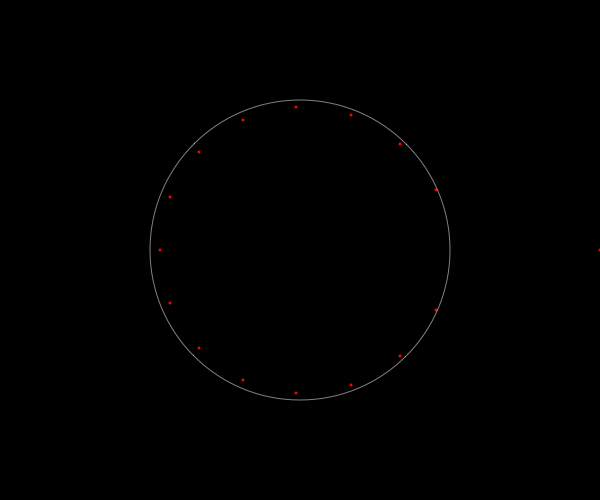The sequence above shows subsets of the Derbyshire set of order 16 (i.e. roots of polynomials of order 16 whose coefficients belong to $\{-1,1\}$), with each frame coming from polynomials with a particular sum of coefficients (or equivalently, a particular number of coefficients equal to $1$ rather than $-1$).

It’s interesting that at least some of the “fractal” structure seems to be present within the largest of these subsets, as opposed to needing all of the subsets combined to make it apparent. So I guess something interesting must arise solely from permuting the coefficients, at least when there are roughly equal numbers of $1$s and $-1$s.

Posted by: Greg Egan on December 9, 2009 11:16 AM | Permalink | Reply to this

### Re: This Week’s Finds in Mathematical Physics (Week 285)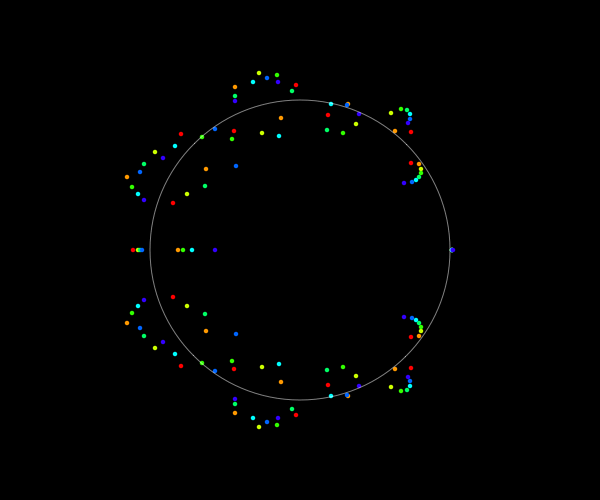Above are the roots of some polynomials with 8 coefficients of 1 and 9 of -1:

$z^{16}+z^{15}+z^{14}+z^{13}+z^{12}+z^{11}+z^{10}+z^9-z^8-z^7-z^6-z^5-z^4-z^3-z^2-z-1$

$z^{16}+z^{15}+z^{14}+z^{13}+z^{12}+z^{11}+z^{10}-z^9+z^8-z^7-z^6-z^5-z^4-z^3-z^2-z-1$

$z^{16}+z^{15}+z^{14}+z^{13}+z^{12}+z^{11}+z^{10}-z^9-z^8+z^7-z^6-z^5-z^4-z^3-z^2-z-1$

$z^{16}+z^{15}+z^{14}+z^{13}+z^{12}+z^{11}+z^{10}-z^9-z^8-z^7+z^6-z^5-z^4-z^3-z^2-z-1$

$z^{16}+z^{15}+z^{14}+z^{13}+z^{12}+z^{11}+z^{10}-z^9-z^8-z^7-z^6+z^5-z^4-z^3-z^2-z-1$

$z^{16}+z^{15}+z^{14}+z^{13}+z^{12}+z^{11}+z^{10}-z^9-z^8-z^7-z^6-z^5+z^4-z^3-z^2-z-1$

$z^{16}+z^{15}+z^{14}+z^{13}+z^{12}+z^{11}+z^{10}-z^9-z^8-z^7-z^6-z^5-z^4+z^3-z^2-z-1$

$z^{16}+z^{15}+z^{14}+z^{13}+z^{12}+z^{11}+z^{10}-z^9-z^8-z^7-z^6-z^5-z^4-z^3+z^2-z-1$

$z^{16}+z^{15}+z^{14}+z^{13}+z^{12}+z^{11}+z^{10}-z^9-z^8-z^7-z^6-z^5-z^4-z^3-z^2+z-1$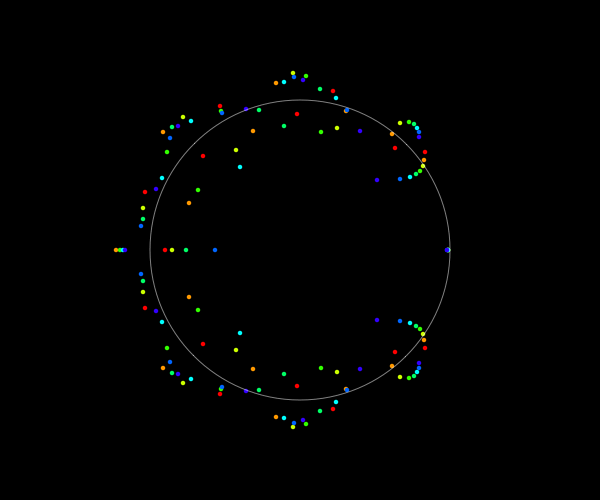Above are the roots of some polynomials with 9 coefficients of 1 and 8 of -1:

$z^{16}+z^{15}+z^{14}+z^{13}+z^{12}+z^{11}+z^{10}+z^9+z^8-z^7-z^6-z^5-z^4-z^3-z^2-z-1$

$z^{16}+z^{15}+z^{14}+z^{13}+z^{12}+z^{11}+z^{10}+z^9-z^8+z^7-z^6-z^5-z^4-z^3-z^2-z-1$

$z^{16}+z^{15}+z^{14}+z^{13}+z^{12}+z^{11}+z^{10}+z^9-z^8-z^7+z^6-z^5-z^4-z^3-z^2-z-1$

$z^{16}+z^{15}+z^{14}+z^{13}+z^{12}+z^{11}+z^{10}+z^9-z^8-z^7-z^6+z^5-z^4-z^3-z^2-z-1$

$z^{16}+z^{15}+z^{14}+z^{13}+z^{12}+z^{11}+z^{10}+z^9-z^8-z^7-z^6-z^5+z^4-z^3-z^2-z-1$

$z^{16}+z^{15}+z^{14}+z^{13}+z^{12}+z^{11}+z^{10}+z^9-z^8-z^7-z^6-z^5-z^4+z^3-z^2-z-1$

$z^{16}+z^{15}+z^{14}+z^{13}+z^{12}+z^{11}+z^{10}+z^9-z^8-z^7-z^6-z^5-z^4-z^3+z^2-z-1$

$z^{16}+z^{15}+z^{14}+z^{13}+z^{12}+z^{11}+z^{10}+z^9-z^8-z^7-z^6-z^5-z^4-z^3-z^2+z-1$

$z^{16}+z^{15}+z^{14}+z^{13}+z^{12}+z^{11}+z^{10}+z^9-z^8-z^7-z^6-z^5-z^4-z^3-z^2-z+1$Above are the roots of some polynomials with 10 coefficients of 1 and 7 of -1:

$z^{16}+z^{15}+z^{14}+z^{13}+z^{12}+z^{11}+z^{10}+z^9+z^8+z^7-z^6-z^5-z^4-z^3-z^2-z-1$

$z^{16}+z^{15}+z^{14}+z^{13}+z^{12}+z^{11}+z^{10}+z^9+z^8-z^7+z^6-z^5-z^4-z^3-z^2-z-1$

$z^{16}+z^{15}+z^{14}+z^{13}+z^{12}+z^{11}+z^{10}+z^9+z^8-z^7-z^6+z^5-z^4-z^3-z^2-z-1$

$z^{16}+z^{15}+z^{14}+z^{13}+z^{12}+z^{11}+z^{10}+z^9+z^8-z^7-z^6-z^5+z^4-z^3-z^2-z-1$

$z^{16}+z^{15}+z^{14}+z^{13}+z^{12}+z^{11}+z^{10}+z^9+z^8-z^7-z^6-z^5-z^4+z^3-z^2-z-1$

$z^{16}+z^{15}+z^{14}+z^{13}+z^{12}+z^{11}+z^{10}+z^9+z^8-z^7-z^6-z^5-z^4-z^3+z^2-z-1$

$z^{16}+z^{15}+z^{14}+z^{13}+z^{12}+z^{11}+z^{10}+z^9+z^8-z^7-z^6-z^5-z^4-z^3-z^2+z-1$

$z^{16}+z^{15}+z^{14}+z^{13}+z^{12}+z^{11}+z^{10}+z^9+z^8-z^7-z^6-z^5-z^4-z^3-z^2-z+1$

$z^{16}+z^{15}+z^{14}+z^{13}+z^{12}+z^{11}+z^{10}+z^9-z^8+z^7+z^6-z^5-z^4-z^3-z^2-z-1$

The general effect seems to be that as you shift the coefficient that gets the plus sign you can move the roots around various curves, but I can’t see any simple formula for what’s going on here.

Posted by: Greg Egan on December 9, 2009 12:54 PM | Permalink | Reply to this

### Re: This Week’s Finds in Mathematical Physics (Week 285)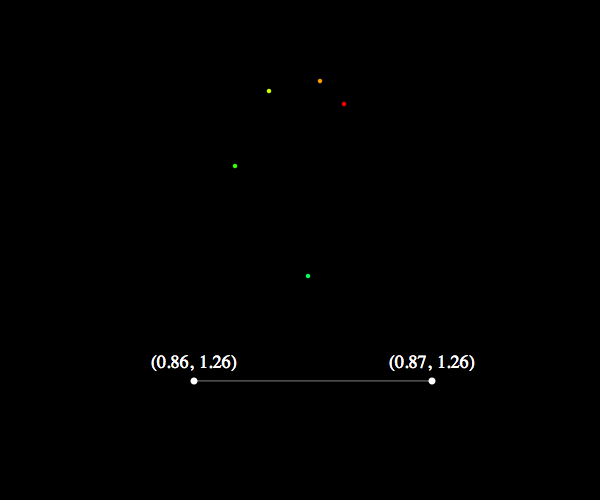One more example of a tight spiral of roots as we shift a sign along the coefficients. These five roots are from the polynomials:

$z^{16}-z^{15}+z^{14}+z^{13}+z^{12}-z^{11}-z^{10}+z^9+z^8+z^7+z^6+z^5-z^4-z^3-z^2-z+1$

$z^{16}-z^{15}+z^{14}+z^{13}+z^{12}-z^{11}-z^{10}+z^9+z^8+z^7+z^6+z^5-z^4-z^3-z^2+z-1$

$z^{16}-z^{15}+z^{14}+z^{13}+z^{12}-z^{11}-z^{10}+z^9+z^8+z^7+z^6+z^5-z^4-z^3+z^2-z-1$

$z^{16}-z^{15}+z^{14}+z^{13}+z^{12}-z^{11}-z^{10}+z^9+z^8+z^7+z^6+z^5-z^4+z^3-z^2-z-1$

$z^{16}-z^{15}+z^{14}+z^{13}+z^{12}-z^{11}-z^{10}+z^9+z^8+z^7+z^6+z^5+z^4-z^3-z^2-z-1$

Posted by: Greg Egan on December 9, 2009 2:25 PM | Permalink | Reply to this

### Re: This Week’s Finds in Mathematical Physics (Week 285)

Hi Egan, I have a request. Can you plot the roots in a binary fashion, that is, instead of {1;-1}, could you plot {1;0}, and start a simple count from 1 to 2^10, sequentially, in binary?

Posted by: Daniel de França MTd2 on December 9, 2009 3:45 PM | Permalink | Reply to this

### Re: This Week’s Finds in Mathematical Physics (Week 285)

This idea of “binary” isn’t far off of how I’ve been enumerating all possible polynomials. For example, the picture of mine John posted had coefficients of the cube roots of unity. To enumerate these, I basically wrote down all the strings in trinary of a certain length and then replaced the usual numerals (0, 1, 2) with the numerals (1, e^{2pi i /3}, e^{4pi i /3}. This generalizes in a straightforward way a method of taking a list of n coefficients and enumerating all the possible polynomials.

I have some more ideas for assigning “numerals”. Right now I’m generating a picture of the roots for polynomials whose coefficients are like the quantum numbers of the baryon decuplet. The hypercharge is the imaginary part of the coefficient, the third isospin component is the real part. We’ll see how they go, but I think they’ll probably look interesting.

I should also note, as long as I’m posting, that I am plotting ALL the roots of ALL the polynomials up to degree d, not just the polynomials of degree d. Indeed, my coloring scheme is by degree.

As a side note, my favorite thing I’ve seen so far is in the fifth roots of unity, along the negative real axis. There’s a weird little star, embedded in an empty circle, whose outer boundary is roughly 5-fold symmetric too. You can see it really clearly in the “inverse” structure nearly all the way out on the negative real axis.

It has analogs in some (maybe all?) of the other degrees, but it’s not as clear in other instances.

Another comment I should make is that the pictures that don’t correspond to Z2 all have to be plotted from -2 to 2 instead of from -phi to phi. I’m betting there’s a straightforward reason for this (eg. the coefficient on the sub-leading term is like the sum of the roots, or something to this effect), but until the semester really ends, there’s no hope of me trying to show the reason for this.

Posted by: Evan Berkowitz on December 16, 2009 5:39 AM | Permalink | Reply to this

### Re: This Week’s Finds in Mathematical Physics (Week 285)These are the roots of polynomials with coefficients drawn from $\{0,1\}$, coloured by the integer whose binary digits match the sequence of coefficients (which is just $P(2)$, if you think about it). The hue shifts from red for the lowest $P(2)$ to blue for the highest values; this image ranges from $P(2)=2$ (i.e. $P(z)=z$) to $P(2)=1023$ (i.e. $P(z)=z^9+z^8+...+z+1$).

Posted by: Greg Egan on December 9, 2009 11:08 PM | Permalink | Reply to this

### Re: This Week’s Finds in Mathematical Physics (Week 285)

Here’s a very rough explanation as to why we should expect “fractal” spirals to appear in these root sets.

Suppose we have a polynomial in our set, $R(z)$, with a root at $z=r$. And suppose there’s a family of other polynomials in our set, which are all related to $R(z)$ by:

$P_n(z)=R(z)+a z^n$

where $a$ is a constant. A Taylor expansion about $z=r$ gives:

$P_n(r+w)\approx a r^n + (n a r^{n-1} + R'(r))w$

So (in so far as the Taylor expansion is useful for approximating roots of $P_n(z)$ near $r$), we can expect roots $r_n$ of approximately:

$r_n\approx r - \frac{a r^n}{n a r^{n-1} + R'(r)}$

If $R'(r)$ dominates $n a r^{n-1}$, we’d expect a spiral pattern centred on the original root $r$:

$r_n\approx r - b r^n$

where $b=\frac{a}{R'(r)}$.

Of course this is all pretty crude and hand-wavy, but qualitatively it says: expect sometimes to see roots occur in spirals around other roots, at scales that can be expected to shrink as $R'(r)$ gets large.

Posted by: Greg Egan on December 9, 2009 10:39 PM | Permalink | Reply to this

### Re: This Week’s Finds in Mathematical Physics (Week 285)

0 1 2 4 5 0 2 4 5 0 2 5

The diagram above shows some roots related in a kind of tree structure based on the addition of monomials to a reference polynomial:

$R(z)=z^{24}-z^{23}+z^{22}+z^{21}-z^{20}+z^{19}+z^{18}-z^{17}-z^{16}-z^{15}-z^{14}+z^{13}+z^{12}-z^{11}+z^{10}$ $+z^9+ z^8+z^7+z^6-z^5-z^4+z^3-z^2-z-1$

A root of $R(z)$ is given in black. The points in red marked with an integer $n$ are roots of $R(z)+2z^n$; those in green are roots of $(R(z)+2z)+2z^n$, and those in blue are roots of $(R(z)+2z+2z^4)+2z^n$.

This structure is broadly as I predicted from the Taylor series approximation, but although it explains why there are lots of roughly congruent spirals nestled together, it doesn’t really explain why there are smaller spirals attached.

Posted by: Greg Egan on December 10, 2009 4:58 AM | Permalink | Reply to this

### Re: This Week’s Finds in Mathematical Physics (Week 285)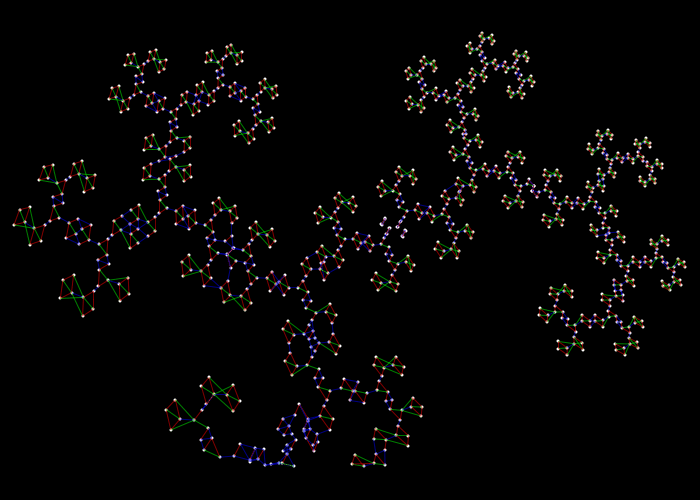Here’s part of a “dragon” for the order 16 Derbyshire set, with every root joined to at least three close neighbours.

The edges in the graph are coloured red if the polynomials whose roots lie at the vertices differ by a single term; green, if they differ by two terms; blue, if they differ by three or more terms.

There’s clearly something more subtle going on than the spirals of roots I identified previously, but I can’t yet see how to link the algebraic structure here with the geometry.

Posted by: Greg Egan on December 10, 2009 7:09 AM | Permalink | Reply to this

### Re: This Week’s Finds in Mathematical Physics (Week 285)

Fix $z$ with $\|z\|<1$, and consider the set of all signum polynomials evaluated at $z$. This is a set closed under the maps $x\mapsto 1+zx$ and $x\mapsto 1-zx$; check that this makes a dragon $D(z)$! Furthermore, $z$ is in Derbyshire’s picture iff $0$ is in our dragon!! So if $D(z)$ were what you’d call a Julia set, then Derbyshire’s picture $\Delta$ is the corresponding Mandelbrot!!!

The map $z\mapsto \overline{D(z)}$ is continuous as a map from the open unit disc to closed subsets of $\mathbb{C}$ in the Hausdorff metric; hence so is $z\mapsto \mathrm{Distance}(0,D(z))$; and in particular its preimage near a point $z\in \Delta$ should look an awful lot like $D(z)$. This is in good analogy to the Mandelbrot/Julia set boundary renormalization.

What think we?

Posted by: some guy on the street on December 10, 2009 7:03 PM | Permalink | Reply to this

### Re: This Week’s Finds in Mathematical Physics (Week 285)

All this stuff is really cool! Unfortunately I’m spending today grading final exams, so I can’t come out and play.Posted by: John Baez on December 10, 2009 7:17 PM | Permalink | Reply to this

### Re: This Week’s Finds in Mathematical Physics (Week 285)

Great, “some guy on the street” seems to have nailed it!

To spell things out a bit more slowly, if we define two maps:

$f_{z,+}(x)=1+z x$ $f_{z,-}(x)=1-z x$

then we can generate all the Littlewood polynomials (polynomials with coefficients $\pm 1$) of degree $d$ in $z$ as some sequence of $d+1$ compositions of our two maps, starting at $x=0$ (well, modulo an overall sign, as the constant term we get is always $1$ and never $-1$). For example:

$f_{z,+}(f_{z,-}(f_{z,-}(0)))=1+(1-z)z=1+z-z^2$

Now, if we use our two maps for an Iterated Function System, starting with $x=0$ then applying some random sequence of one map or the other, the kind of result we get will depend on our choice of $z$. Sometimes this will look like a dragon: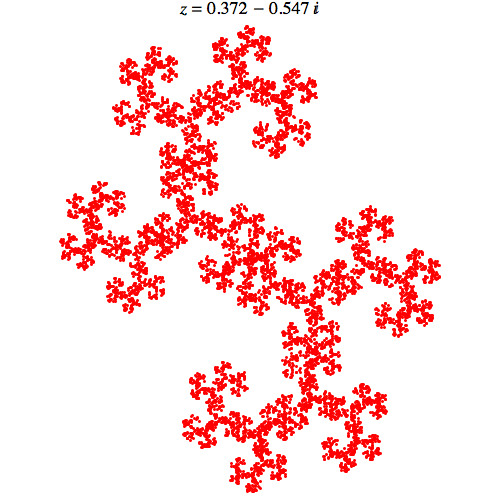Sometimes it will look like a feather: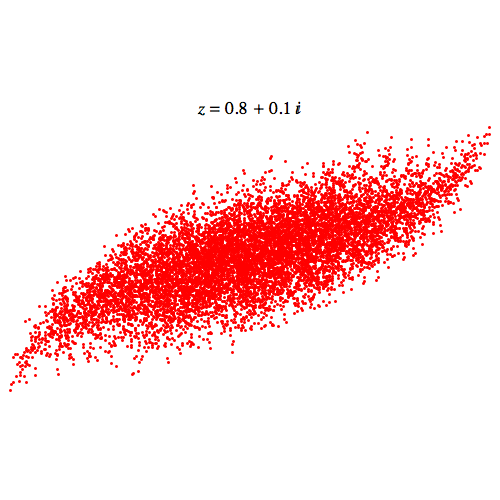These are like Julia sets for a probabilistic dynamic system where you iterate with either one map or the other, and Derbyshire’s set of roots is like the corresponding Mandelbrot set, where $z$ being a root of a Littlewood polynomial means that some sequence of the maps will bring you back to zero.

Posted by: Greg Egan on December 10, 2009 11:10 PM | Permalink | Reply to this

### Re: This Week’s Finds in Mathematical Physics (Week 285)

So, let`s see if this really works. Try to find the spirals on the imaginary axis.

Posted by: Daniel de Franca MTd2 on December 11, 2009 12:38 AM | Permalink | Reply to this

### Re: This Week’s Finds in Mathematical Physics (Week 285)

They might look something like this

rectangle with filaments

in case that helps. This is $D(.001+.71i)$, for what it’s worth. Not much; but it also suggests something more of why the picture gets cloudy towards the unit circle.

Posted by: some guy on the street on December 11, 2009 2:43 AM | Permalink | Reply to this

### Re: This Week’s Finds in Mathematical Physics (Week 285)

I get something similar.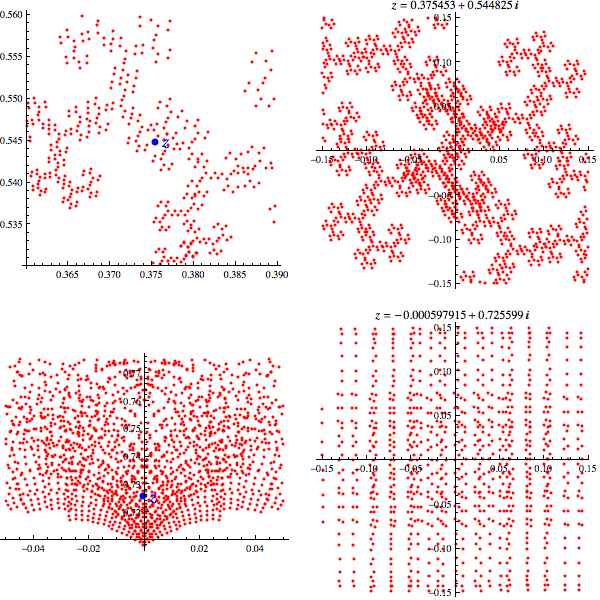The pictures on the left are roots of the Littlewood polynomials of degree 16, near $z$; those on the right are those images of $z$ that lie near $0$ under the same polynomials. In the second row, there’s still a clear qualitative resemblance, though the cusps seem to be complicating things. This resemblance isn’t expected to work perfectly, absolutely everywhere.

Posted by: Greg Egan on December 11, 2009 4:52 AM | Permalink | Reply to this

### Re: This Week’s Finds in Mathematical Physics (Week 285)

For curiosity, I had sage plot the least absolute value of Derbyshire’s polynomials up to degree 7, (if I’ve counted right); and you can sort-of see some of the dragon shapes starting to shape-up around the edges:otherwise, it’s not a great picture. I asked for 300x300 resolution, but I don’t believe this shows that much.

Incidentally, does anyone know how to ask sage to just plot colours, or must I get them indirectly from a 3d-plot?

Posted by: some guy on the street on December 10, 2009 2:56 AM | Permalink | Reply to this

### Re: This Week’s Finds in Mathematical Physics (Week 285)

Here’s a heuristic way of understanding why the set of roots of the Littlewood polynomials that lie near $z$ resembles the set of images of $z$ under the same Littlewood polynomials.

The image of $z$ under all the Littlewood polynomials is the same as iterating with every possible composition of the maps $f_{z,+}(x)=1+z x$ and $f_{z,-}(x)=1-z x$, applied to a “seed point” of $0$. If we get a nice fractal from that Iterated Function System, then if we zoom in close to the origin we should see something qualitatively the same.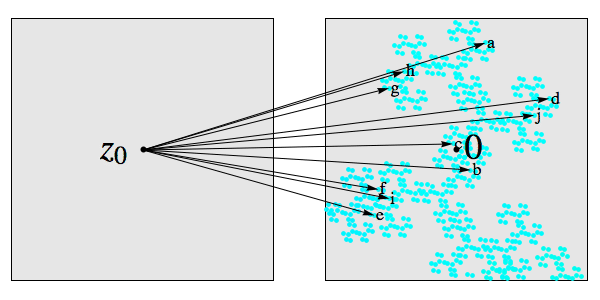In the picture above, $z$ is mapped to a set of points near $0$ by some subset of the Littlewood polynomials. This image is just a subset of the image of $z$ under all the polynomials, but because the complete image is a fractal, the subset near the origin will resemble it.

But if we grab all these arrows and squeeze their tips together so that they all map precisely to $0$ – and if we’re working in a small enough neighbourhood of $0$ that the arrows don’t really change much as we move them – the pattern that imposes on the tails of the arrows will look a lot like the original pattern: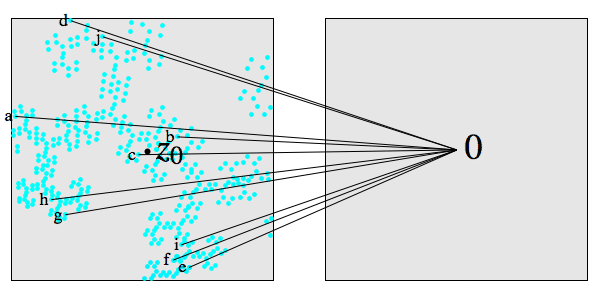Posted by: Greg Egan on December 11, 2009 4:10 AM | Permalink | Reply to this

### Re: This Week’s Finds in Mathematical Physics (Week 285)

One perhaps useful trick here, I think, might be to note that since similar polynomials that land “near” zero for a given region around some $z_0$ not only have similar values for $z$ near $z_0$,but also have similar derivatives: if $P$ and $Q$ differ only for terms of degree at least $n$, then so do their derivatives, so for $|z_0|<1$, these higher-order terms are less significant.

(It might be fruitful to think of what some guy calls $D(z)$ as a map from the product of the unit disc and the Cantor set, in the obvious way; then you all know how similar polynomials are.)

So if $P(z_0)$ is zero, and $Q$ is similar to $P$, then the correction $\delta$ solving $Q(z_0+\delta)=0$ is very nearly $-Q(z_0)/P'(z_0)$.

So, where things might start to get weird is if several dissimlar polynomials are small at $z_0$; e.g. in case $z=\sqrt{-\frac{1}{2}}$, we have $1+z+z^2+z^3-z^4-z^5 ++-- \cdots$ tending to zero, as well as $1-z+z^2-z^3-z^4+z^5+z^6--++\cdots$ So there are at least two different ways of moving off this $z_0$ into widely separated branches of the Cantor set while still getting new roots; and that neatly describes how near that cuspy point we have what looks like two grids intersecting at a funny angle. The angle probably has something to do with $1+z+\frac{z^2+z^3}{1+z^2} \qquad vs. \qquad 1-z+\frac{z^2-z^3}{1+z^2}$

Oh, can someone tell me if there is a stack lurking here? I’ve still got a hazy cloud between me and stacks, but this family of $D(z)$s sounds like the kinds of examples I’ve seen mentioned for the uninitiate.

Posted by: Jesse McKeown on December 12, 2009 3:40 AM | Permalink | Reply to this

### Re: This Week’s Finds in Mathematical Physics (Week 285)

that neatly describes how near that cuspy point we have what looks like two grids intersecting at a funny angle

Very nice!

Posted by: Greg Egan on December 12, 2009 4:08 AM | Permalink | Reply to this

### Algebraics avoid eachother

Well, that’s all very nice. Someone mentioned holes, as well, though. Let’s have a try!

I’m afraid some guy has shown that the hole around $1$ has to shrink by about 1/2 as “soon” as you work out the roots for all the Littlewood polynomials to degree $32$; so pretty much all of those on the circle will get flooded eventually. Some of the holes off the circle might just survive, though, because algebraics avoid eachother. Of course, everybody knows that rationals avoid irrational algebraics. Here’s a kludge to extend this result.

Suppose $P$ and $Q$ are distinct irreducible integer polynomials of degrees $p,q$, and write $\lambda_i$ for the roots of $P$, $\mu_j$ for the roots of $Q$. Then we can write down (ugly) upper bounds $L$ and $M$ for $|\lambda_i|$ and $|\mu_j|$ in terms of the coefficients of $P$ and $Q$, respectively. I believe

$\max (1,\sum |e_i|)$

will do, for $e_i$ the (rational) normalized coefficients. Now note that

$\prod_i Q(\lambda_i)$

is a symmetric function of the roots of $P$, and hence is (nonzero!) rational; we can even estimate it from below, by writing the result $\delta$ as an (even uglier) expression in the coefficients of $P$ and bounding its height from above. (If $P$ is monic, then this is easier, because it’s an integer). On the other hand,

$\prod_i Q(\lambda_i) = \prod_{i,j} Q_q (\lambda_i - \mu_j)$

where each factor is at most $L+M$; in Koosis’ words, “what can’t be too large can’t be too small” (although he usually means harmonic functions…). In this case we have

$|\lambda_i - \mu_j| \geq \frac{\delta}{Q_q^p(L+M)^{pq-1}} .$

OK, it’s not a spectacular lower bound. We don’t even reproduce Liouville’s estimate restricting to the case $q=1$. But it is a lower bound, quantitative as anyone could like, and I’m sure some clever lass or fellow out there will (or has) come up with something better.

Posted by: Jesse McKeown on December 11, 2009 4:46 AM | Permalink | Reply to this

### Re: Algebraics avoid eachother

We don’t even reproduce Liouville’s estimate restricting to the case $q=1$.

Silly me, of course we do reproduce at least the power law. Some days I can’t read my own typing! The constant won’t be great, but we are talking about all the roots of $P$ and $Q$ now; and also we haven’t even mentioned derivatives of $Q$ (or $P$…)!

Posted by: Jesse McKeown on December 12, 2009 8:13 PM | Permalink | Reply to this

### Re: This Week’s Finds in Mathematical Physics (Week 285)

Evan Berkowitz has also been making pictures!

For example, here’s his picture of all roots of all polynomials of degree $\le 9$ with coefficients that are cube roots of unity:You can see more pictures by Berkowitz catalogued here. The file ‘C$n$-$k$-$p$.png’ shows all roots of all polynomials of degree $\le k$ whose coefficients are $n$th roots of unity, in an image that is $p \times p$ pixels in size.

When $n = 2$ we’re back to the ‘Derbyshire sets’, which consist of all roots of all polynomials of degree $\le k$ with coefficients that are $+1$ or $-1$.

Posted by: John Baez on December 11, 2009 8:21 AM | Permalink | Reply to this

### Re: This Week’s Finds in Mathematical Physics (Week 285)

John, on the TWF page you say:

[Sam Derbyshire] made a high-resolution plot by computing all the roots of all polynomials of this sort having degree 24.

but then here you say:

Sam’s picture shows all roots of all polynomials of degree $\le 24$

I suspect both sets would look extremely similar, but just for the record, are Sam’s pictures all degrees up to and including 24, or just degree 24 alone?

Posted by: Greg Egan on December 12, 2009 3:14 AM | Permalink | Reply to this

### Re: This Week’s Finds in Mathematical Physics (Week 285)

Sorry. Degree $\le 24$. Here is what Sam actually told me:

My favourite kind was taking polynomials with coefficients only +1 or -1 (0 not allowed), so I did a high resolution version of it. I’ve uploaded it here [see below].

The file is rather big (about 23 000 × 16 000 pixels), it took about 4 days for Mathematica to generate the coordinates of the roots (it ended up being about 5 GB of data), then I used some Java programs to put that into an image.

In this case I took the degree of the polynomials to be 24 - that’s about 400 000 000 roots! The colouring just shows density of roots around that point, from dark red to yellow to white.

The count of roots only makes sense if he meant all polynomials of degree $\le 24$, since $24 \times 2^{24} = 402,653,184$. I’ll fix This Week’s Finds to make this more clear.

Sam uploaded his huge file on a certain commercial site, but it may not stay there… this location is more stable:

Posted by: John Baez on December 12, 2009 3:38 AM | Permalink | Reply to this

### Re: This Week’s Finds in Mathematical Physics (Week 285)

The count of roots [about 400 000 000] only makes sense if he meant all polynomials of degree $\le 24$, since $24\times 2^{24}=402,653,184$.

Huh? Your calculation is for the count of roots of polynomials of degree exactly 24, so haven’t you shown that Sam’s picture is for degree 24 only?

Posted by: Greg Egan on December 12, 2009 4:05 AM | Permalink | Reply to this

### Re: This Week’s Finds in Mathematical Physics (Week 285)

Oh, you’re right. I was forgetting that a polynomial of degree $\le 24$ has 25 coefficients. For the same annoying reason an $n$ category is built from $n+1$ kinds of things: objects, 1-morphisms, 2-morphisms, on up to $n$-morphisms. Zero is the first natural number! I always trip over that fencepost. I was imagining 24 coefficients and about 24 roots per polynomial. (Not exactly, of course.)

So I guess he really meant the degree was exactly 24. Maybe I’ll ask him.

I must admit I never dreamed that he was restricting attention to polynomials of degree exactly $n$. But now that I think about it, this is perfectly sensible if you’re studying polynomials whose coefficients are all $1$ or $-1$. A degree $\lt n$ polynomial, thought of as a degenerate degree-$n$ polynomial, would have some coefficients equal to zero!

Posted by: John Baez on December 12, 2009 6:46 AM | Permalink | Reply to this

### Re: This Week’s Finds in Mathematical Physics (Week 285)

Here’s another possible heuristic argument for why some of these root pictures have “holes” at roots of unity. (I’m not sure whether/how it’s related to the “algebraic numbers avoid each other” phenomenon.)

Consider looking for polynomials which have a root near a specific value x, in order to estimate the density of roots near x, in one of these pictures (with some limit on degree and coefficients of the polynomial). First grab lots of polynomials and evaluate them at exactly x; keep only the ones whose values are close to 0. For each of these polynomials, very near x it’s roughly linear, so it probably has one exact root near x, so the number of polynomials we kept estimates the number of roots near x.

If x is a root of unity, a lot of its powers will be equal, so a lot of the polynomials we keep will be equal (especially in the limit of high degree), so there are many fewer distinct polynomials that we keep. To put it another way, the polynomials we keep are those in some neighborhood in a “lattice” (indexed by the polynomials coefficients), but for x a pth root of unity, this lattice has only p dimensions; otherwise it has d dimensions if we go up to degree d.

Posted by: Bruce Smith on December 13, 2009 2:50 AM | Permalink | Reply to this

### Re: This Week’s Finds in Mathematical Physics (Week 285)

How can these beautiful pictures of roots increase our understanding of Galois theory?

Posted by: sandcat on December 14, 2009 9:41 AM | Permalink | Reply to this

### Re: This Week’s Finds in Mathematical Physics (Week 285)

Interesting question! These pictures have a large group of symmetries, namely the absolute Galois group of the rationals, $Gal(\overline{\mathbb{Q}}/\mathbb{Q})$. However, I don’t think these symmetries are visible in the pictures, except for complex conjugation. I’d love to be proved wrong.

Posted by: John Baez on December 29, 2009 7:35 PM | Permalink | Reply to this

### Re: This Week’s Finds in Mathematical Physics (Week 285)

Scientific American has now cashed in on the beauty of roots:

Posted by: John Baez on December 29, 2009 6:43 PM | Permalink | Reply to this

### Re: This Week’s Finds in Mathematical Physics (Week 285)

There’s an interesting video of polynomial roots on Youtube. A more systematic approach might yield videos that are very informative as well as beautiful.

Posted by: John Baez on January 9, 2010 11:13 PM | Permalink | Reply to this

### Re: This Week’s Finds in Mathematical Physics (Week 285)

I’ve made some more interesting renderings. As a generalization of what I’d done before, which was using the roots of unity as coefficients, I figured that if I had two circles on which to put the coefficients, things might get even more interesting. So, arbitrarily, I picked circles of radius GoldenRatio and 1/GoldenRatio, and put down coefficients. I picked the golden ratio because it came up a couple of times in this context previously.

I rendered one or two images like this for four evenly spaced coefficients on each circle:

GoldenRatio e^{2 pi i n / 4} and GoldenRatio^{-1} e^{2 pi i n / 4}.

The image is nice, but what came next is (I think) even more interesting. I let the inner roots rotate with respect to the outer roots. So, the set of 8 coefficients became

GoldenRatio e^{2 pi i n / 4} and GoldenRatio^{-1} e^{(2 pi i n / 4) + i theta}

Then, each theta defines a set of coefficients, and you can find the roots of those polynomials. Moreover, you can let theta increase continuously, and get a movie! As you might imagine, each one takes some time to compute and render, so I’ve only made a few so far.

Here are the movies:
Two coefficients on each circle: http://www.youtube.com/watch?v=1yWu9BtNk8M
Three coefficients on each circle: http://www.youtube.com/watch?v=YWMZJytSUDU
Four coefficients on each circle: http://www.youtube.com/watch?v=e5lwlJaFcnI
Five coefficients on each circle: http://www.youtube.com/watch?v=YqIeDslPNgs

Their time step is set so that it takes 60 frames to go 2 pi / n where n is the number of coefficients on each circle. After 120 frames, I add the polynomials of the next degree. It should be relatively clear when that happens.

The next thing to look at, I think, is to mix the number of coefficients on each circle (so, let the inner circle have 3 coefficients but the outer circle have 4, for example). I also think it would be interesting to try to classify the kinds of trajectories the roots make (the routes of the roots!). Something else might be to try making 3D plots of these objects, using my time direction as a spatial direction.

Posted by: Evan Berkowitz on January 18, 2010 5:22 AM | Permalink | Reply to this

### Re: This Week’s Finds in Mathematical Physics (Week 285)

I’ve made some additional movies, and posted one to Youtube. This movie is more directly connected with the k-fold symmetric images I was rendering (here).

The coefficients for the polynomials that generated those pictures were picked to be

exp(i n pi /4) n=0,1,2,3,

for the 4-fold symmetric picture (as an example). Instead, pick the coefficients to be

exp(i n theta /4) n=0,1,2,3

when theta=0, all the coefficients are 1 and thus the roots lie on the unit circle. When theta=2pi, then the coefficients are those for the static 4-fold symmetric one. But then something nice happens.

When theta=8pi/3, the coefficients happen to be the same as the coefficients for the static 3-fold symmetric picture, except that one of the coefficients has multiplicity 2 (which you of course cannot see in the plot of the roots!)

When theta=4 pi, the coefficients are +/-1, giving the original Derbyshire set.

Then, as theta increases further, you get a three-fold and four-fold symmetric set again, after which all of the roots return to the unit circle.

I rendered this for polynomials up to degree 4, with theta going linearly with time, from theta=0 to theta=4*2pi. The movie is here:

It’s best watched through completely one time, and then interrupted with pauses at critical moments.

Of course, there’s no reason to work with just four coefficients. Working with five will make the the five, four, three, two -fold symmetric images show up. In principle, if you pick an integer and make this sort of movie, all of the static sets whose symmetry is less than or equal to that integer will show up for some theta along the way.

Posted by: Evan Berkowitz on January 27, 2010 4:37 AM | Permalink | Reply to this

### Re: This Week’s Finds in Mathematical Physics (Week 285)

Whoops! An actual hyperlink to the video:

As always, the different colored dots are roots of polynomials of differing degrees.

Posted by: Evan Berkowitz on January 27, 2010 4:42 AM | Permalink | Reply to this

### Re: This Week’s Finds in Mathematical Physics (Week 285)

Here’s a video with eight coefficients

e^{i n theta /8} n=0…7

with polynomials up to degree 3. You can really see what’s going on in terms of the trajectories, the different degrees, etc.